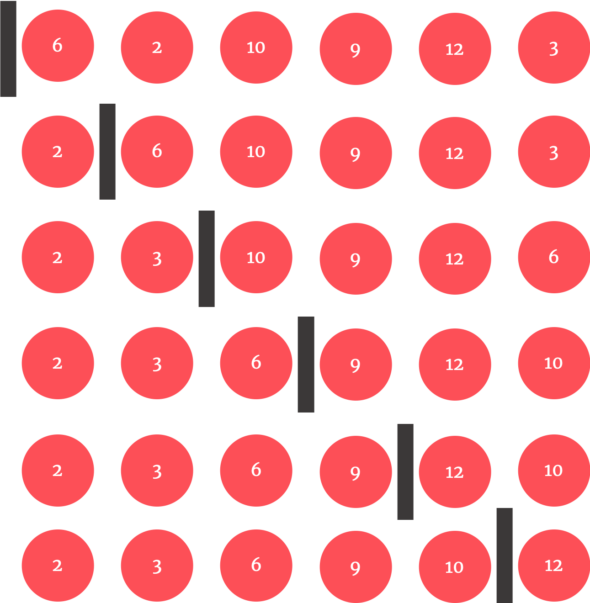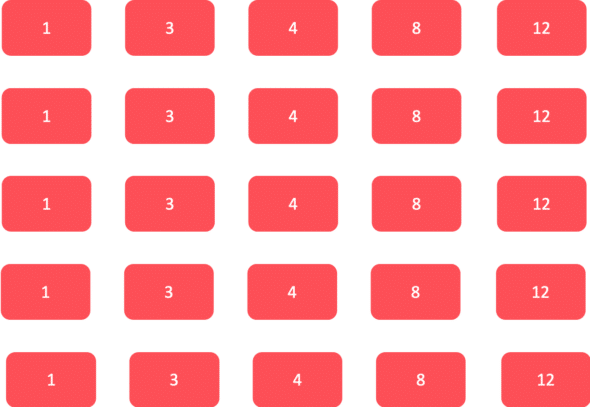# Computer Science for Middle Schoolers – BOGO Sort, Insertion Sort, and Bubble Sort

### Article summary

Once every couple of months, I get an opportunity to teach middle schoolers/high schoolers about computer science.

Computer science and software development are very broad disciplines, so I could cover anything from web styling to computer networks. Among all of those possible topics, I find that I like to work on sorting algorithms.

## Rationale & Setup

Sorting is something all students have experienced, so the problem is pretty well-defined. In essence, we are asking, “How could we take a bunch of numbers that are out of order and put them in order?”

Sorting algorithms introduce some core concepts of computer science: time complexity (if the length of items in the list is n, how long does it take to sort them?) and algorithmic thinking (what are the steps to sort n items and know they will all be sorted?). I also personally like sorting-based activities because they lend themselves well to being interactive.

To prep, I print out some numbers, with one number per piece of paper. If I plan to go over Insertion Sort, I also bring a prop that I can use as a divider.

I start with an introduction about how we are going come up with a way to tell the computer to sort numbers. I ask for some volunteers to come up and help me with the first sorting algorithm. Then, we try out a terrible sorting algorithm, something like BOGO Sort.

## BOGO Sort

BOGO Sort generates a bunch of different combinations of inputs until one of the combinations is sorted.

To replicate this method, I have one student volunteer to be the “randomizer” who is responsible for mixing up all the numbered cards (randomizing the numbers).

The randomizer passes the numbers to a group of volunteers (I usually print out five numbers, so I ask for five volunteers). If the numbers just happen to come out in the same order as the line of volunteers, it means the sort is complete. Otherwise, the randomizer will continue to mix up the number cards and re-distribute them until one of the permutations just happens to be sorted.

As we try again and again for all the numbers to just happen to be sorted, it quickly becomes apparent that BOGO Sort isn’t a good way to get a group of numbers in order.

## Selection Sort

Next, I like to demonstrate either Selection Sort or Insertion Sort. I usually prefer Selection Sort because I find it to be really intuitive. All you have to do is keep bringing the smallest numbers to the beginning of the list.

As a refresher, here is an example of Selection Sort:To run this activity, I get a group of volunteers and give each of them a random number. I put the sort tracker before the first volunteer. We compare all of the numbers in the list to find the smallest one, then we switch the smallest number with the first number in the list. The sort tracker prop moves from a position before the first volunteer to a position after the first volunteer.

Next, we compare all of the numbers after the sort tracker and swap the smallest of those with the first number after the tracker prop. We move the sort tracker prop one to the right and continue this process until all of the elements are sorted. Then I follow up with some discussion questions.

Q: How many things do we have to compare to find the smallest element the first time? The second time?

A: If our list has five elements, the first time we have to compare all five elements. The second time, we know that one of the elements is already sorted, so we only have to compare four elements.

Q: How many times would we have to go through the unsorted section looking for the smallest number before we are done sorting?

A: The answer is one less than the length of the list. If our list is has five elements, we would have to go through the list four times. Once we know the first four elements are sorted, the fifth element must also be sorted.

## Bubble Sort

Bubble Sort goes through the numbers, compares the adjacent numbers, and swaps them if they are in the wrong number. It repeats this through all of the numbers until they are all sorted.

Here is an example of Bubble Sort:

First PassSecond PassThird PassTo demo, I again ask five students to volunteer and give each of them a random number. Starting at the beginning, students standing next to each other compare their numbers. If the numbers are not in the correct order (i.e. the smaller number is not to the left of the larger number) the students switch places. The numbers are not sorted after one pass, so this process is repeated until all of the numbers are sorted.   Classroom Bubble Sort shows this process being done in a classroom.

Once all of the numbers are sorted, we discuss ways we could make the sorting process more efficient.

Q: What does it mean if we go through the numbers and don’t need to swap anything?

A: It means the array is sorted. All the pairs were already ordered correctly and did not need to be swapped.

Q: After going through the numbers once, what do we know about the last number?

A: It is the biggest. This is because each time we compare adjacent numbers, we take the biggest value “with us” and compare it to the next number. So, the biggest number will “bubble up.”

Q: After going through the numbers twice, what do we know?

A: That the last two numbers in the list will be sorted.

Q: After three times, what do we know?

A: That the last three numbers in the list will be sorted.

Q: When going through the list, do we have to compare each number to the other? Have could we compare less numbers?

A: After the first time, we know the last number is sorted, so we don’t need to compare that anymore. After the second time we sort, we know the last two numbers are sorted, so we don’t need to compare to them anymore, etc.

If we have any time left, we re-try the Bubble Sort algorithm, this time using optimizations like the ones we suggested above so students can see that it takes us less time.

Another thing I like to do with remaining time is have the students explain back to me/each other what the sorting algorithms were and pick a favorite. Insertion Sort tends to win the show.

### Related Posts

Conversation
•Chris Davis says:

Thank you for this post! I am preparing a math circle on sorting algorithms for middle school students and I am following your description very closely. I had originally wanted to include a more sophisticated algorithm like merge sort or quick sort, but I found them much harder to describe carefully than bubble sort (which I had actually never heard of before preparing for this math circle). One small typo: In the First pass of your bubble sort example, shouldn’t the 8 and 4 get swapped? Thanks again!

•Lydia Cupery says:

Hello Chris,

I’m glad to hear that you used this post and found it helpful!

You are correct about the bubble sort – the 8 and 4 should have been swapped. I updated this post with that correction. Thanks for catching the typo and commenting.

Best!

Lydia